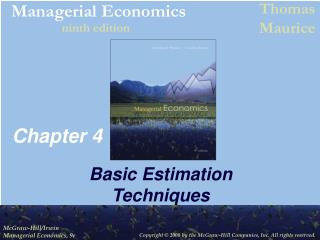DownloadDownload PresentationChapter 4

# Chapter 4

Télécharger la présentation## Chapter 4

- - - - - - - - - - - - - - - - - - - - - - - - - - - E N D - - - - - - - - - - - - - - - - - - - - - - - - - - -
##### Presentation Transcript

1. Chapter 4 Basic Estimation Techniques

2. • Slope parameter (b) gives the change in Y associated with a one-unit change in X, Simple Linear Regression • Simple linear regression model relates dependent variable Y to one independent (or explanatory) variable X

3. • Method of Least Squares • The sample regression line is an estimate of the true regression line

4. ei Sample Regression Line (Figure 4.2) S 70,000 Sales (dollars) • 60,000 • 50,000 • 40,000 • • 30,000 • 20,000 • 10,000 A 10,000 4,000 2,000 8,000 6,000 0 Advertising expenditures (dollars)

5. • Unbiased Estimators • The distribution of values the estimates might take is centered around the true value of the parameter • An estimator is unbiased if its average value (or expected value) is equal to the true value of the parameter

6. Relative Frequency Distribution* (Figure 4.3) 1 4 2 3 8 9 10 5 6 7 0 1 *Also called a probability density function (pdf)

7. Statistical Significance • Must determine if there is sufficient statistical evidence to indicate that Y is truly related to X (i.e., b 0) • Test for statistical significance using t-tests orp-values

8. Performing a t-Test • First determine the level of significance • Probability of finding a parameter estimate to be statistically different from zero when, in fact, it is zero • Probability of a Type I Error • 1 – level of significance = level of confidence

9. Performing a t-Test • Use t-table to choose critical t-value with n – k degrees of freedom for the chosen level of significance • n = number of observations • k = number of parameters estimated

10. Performing a t-Test • If absolute value of t-ratio is greater than the critical t, the parameter estimate is statistically significant

11. Using p-Values • Treat as statistically significant only those parameter estimates with p-values smaller than the maximum acceptable significance level • p-value gives exact level of significance • Also the probability of finding significance when none exists

12. Coefficient of Determination • R2 measures the percentage of total variation in the dependent variable that is explained by the regression equation • Ranges from 0 to 1 • High R2indicates Y and X are highly correlated

13. F-Test • Used to test for significance of overall regression equation • Compare F-statistic to critical F-value from F-table • Two degrees of freedom, n – k & k – 1 • Level of significance • If F-statistic exceeds the critical F, the regression equation overall is statistically significant

14. Multiple Regression • Uses more than one explanatory variable • Coefficient for each explanatory variable measures the change in the dependent variable associated with a one-unit change in that explanatory variable

15. is U-shapedor U -shaped • • • Quadratic Regression Models • Use when curve fitting scatter plot

16. • • • • Log-Linear Regression Models